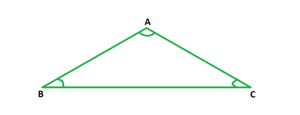Open In App

# How to find the length of an equilateral triangle when the perimeter is given?

In mathematics, the proportions, measurements, dimensions, forms, shapes, and angles of different objects around us are studied under the branch called geometry. Geometry is one of the oldest branches of mathematics and has a very significant application and impact on our daily lives. We are surrounded by innumerable objects in our lives. All these objects possess a certain shape, take up significant space, can be used to store a particular amount of things in them, and can be placed as per different positions. All these factors fall under the scope of geometry. Shapes can be categorized a two- dimensional and three-dimensional.

### Equilateral Triangle

An equilateral triangle has all three sides of equal lengths and all three angles of equal measure, i.e., 60°. The figure below depicts an equilateral triangle ABC with ∠A = ∠B = ∠C = 60°.Perimeter

The perimeter of a triangle is the sum of lengths of all three sides. Since the lengths of sides of an equilateral triangle are equal,

Perimeter of an equilateral side = 3 × Side.

### How to find the length of an equilateral triangle when the perimeter is given?

Solution:

Let the length of side of the equilateral triangle be a units.

Perimeter = a + a + a = 3a units

⇒ a = Perimeter/3.

Thus, the side of an equilateral triangle is one- third of its perimeter.

For example, say the perimeter of an equilateral triangle is 6 cm. Then the length of its side is 6/3 = 2 cm.

### Sample Problems

Question 1. Find the side of an equilateral triangle if its perimeter is 15 cm.

Solution:

Side of an equilateral triangle = Perimeter/ 3

Given: Perimeter = 15 cm

⇒ Side = 15/ 3

= 5 cm

Question 2. Find the side of an equilateral triangle if its perimeter is 18 cm.

Solution:

Side of an equilateral triangle = Perimeter/ 3

Given: Perimeter = 18 cm

⇒ Side = 18/ 3

= 6 cm

Question 3. Find the side of an equilateral triangle if its perimeter is 69 cm.

Solution:

Side of an equilateral triangle = Perimeter/ 3

Given: Perimeter = 69 cm

⇒ Side = 69/ 3

= 23 cm

Question 4. Find the side of an equilateral triangle if its perimeter is 99 cm.

Solution:

Side of an equilateral triangle = Perimeter/ 3

Given: Perimeter = 99 cm

⇒ Side = 99/ 3

= 33 cm

Question 5. Find the side of an equilateral triangle if its perimeter is 45 cm.

Solution:

Side of an equilateral triangle = Perimeter/ 3

Given: Perimeter = 45 cm

⇒ Side = 45/ 3

= 15 cm